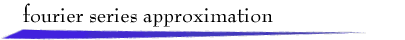For a continuous-time, T-periodic signal x(t), the N-harmonic Fourier series approximation can be written as

 x(t) = a0 + a1 cos (wot + q1) + a2 cos (2wot + q2) + ... + aN cos (Nwot + qN)

where the fundamental frequency wo is 2p /T rad/sec, the amplitude coefficients a1, ..., aN are non-negative, and the radian phase angles satisfy 0 £ q1 , ..., qN < 2p. To explore the Fourier series approximation, select a labeled signal, use the mouse to sketch one period of a signal, or use the mouse to modify a selected signal. Specify the number of harmonics, N, and click "Calculate." The approximation will be shown in red. In addition, the magnitude spectrum (a plot of an vs. n) and phase spectrum (a plot of qn vs. n) are shown. (If the dc-component is negative, a0 < 0, then |a0| is shown in the magnitude spectrum and an angle of p radians is shown in the phase spectrum.) To see a table of the coefficients, click "Table."

Suggested Exercises:
1. Sketch a signal that has a large fundamental frequency component, but small small dc-component and small higher harmonics.
2. Sketch a signal that has large dc and fundamental frequency components, but small higher harmonics.
3. Sketch a signal that has small dc and fundamental frequency components, but large second harmonic.
4. Sketch a signal that has a small fundamental frequency component, but large dc component and large second harmonic.
5. Describe how you would construct a signal that has small dc and fundamental frequency components, but large second, third, and fourth harmonics.return to demonstrations page

 Original applet by Steve Crutchfield, update by Hsi Chen Lee.